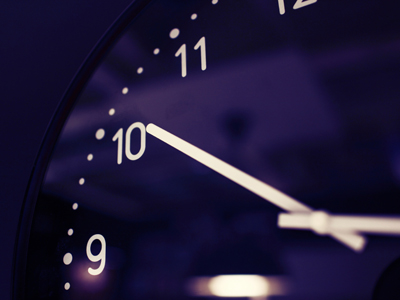What power needs to be added to 10 to give it a value of a million?

# Algebra - Powers 2

This Math quiz is called 'Algebra - Powers 2' and it has been written by teachers to help you if you are studying the subject at middle school. Playing educational quizzes is a fabulous way to learn if you are in the 6th, 7th or 8th grade - aged 11 to 14.

It costs only \$12.50 per month to play this quiz and over 3,500 others that help you with your school work. You can subscribe on the page at Join Us

Remember those pesky little numbers called powers that turned out to be powerful little numbers? Here is another Math quiz on the subject designed to give you some more practice at using algebra and powers together. Good luck!

1.
If a = 4 and b = 9, which is the smallest number from the following?
a4
b3
6a2
9b
9b = 9 x 9 = 81. In a question such as this you have to work out all the answers in order to determine which is correct
2.
b4 - a2 = 621. Given that the value of a is 2, what is a possible value of b?
5
7
9
11
3.
If x = 3, what is the value of x3 + x4 + x5?
27
81
243
351
27 + 81 + 243 = 351
4.
What power needs to be added to 10 to give it a value of a million (1,000,000)?
2
4
6
8
When you are given 10 to the power of something, remember that the power will be the number of zeros that follow the 1. 102 = 100 (2 zeros); 105 = 100,000 (5 zeros)
5.
What is the easiest way to calculate the value of 169?
16 x 9
9 x 16
16 x 16
Use a calculator
By using a calculator we found the answer was 68,719,476,736. Without a calculator it might have taken us a week to work out!
6.
If a = 3 and y = 7, what is the answer to 4a(2y2)?
176
761
1,176
6,711
12 x 98
7.
400 - x3 = 57. What is the value of x?
3
5
7
9
Experiment with different values for x until you find the number that fits
8.
If x = 13 and y = 8, what is the answer to x2 - (20y + 9)?
0
8
13
29
169 - 169 = 0
9.
When a = 11 and b = 15, which of these gives the smallest number?
a2 + 18
10b - 11
b2 - 86
11a
All the incorrect answers have the value of 139
10.
If x = y2, and x = 9 then what is a possible value of y?
3
6
9
12
An easy one to reward you for finishing the quiz! Strictly speaking, it should be plus or minus 3
Author:  Frank Evans